# Intermediate first year maths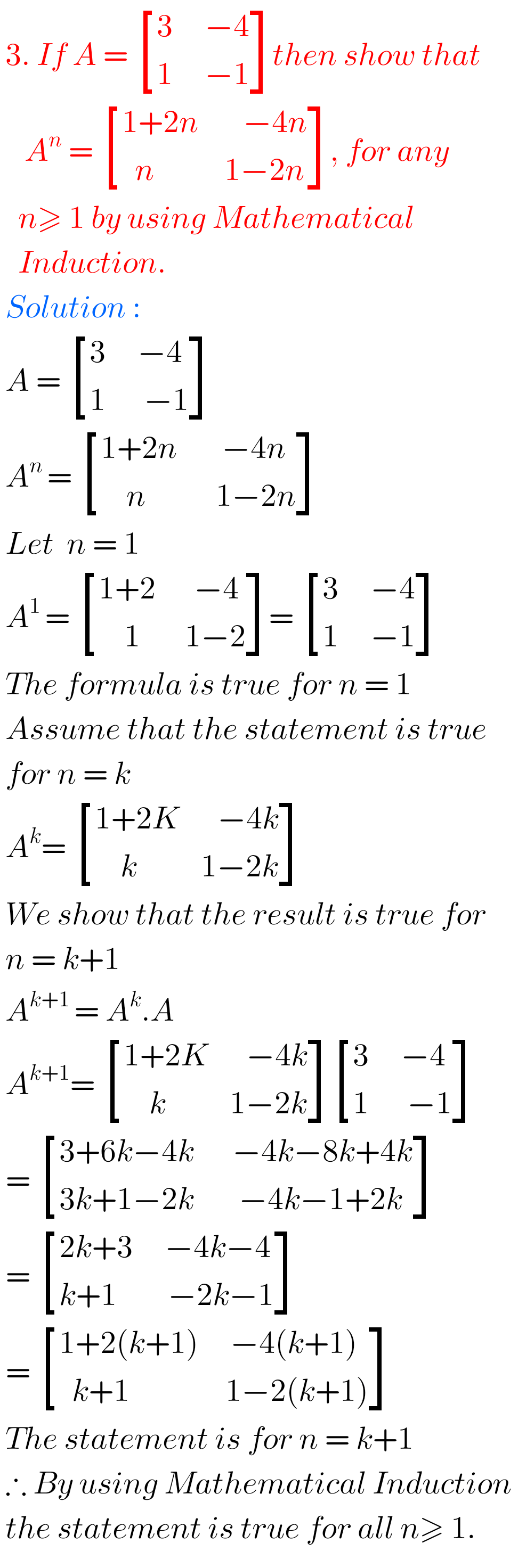## Intermediate Maths Solutions for Exercise 3(b) Matrices

Intermediate Maths Solutions for Exercise 3(b) Matrices Inter Maths 1A text book Exercise 3(b) Matrices solutions are given. These solutions are very easy to understand. Please study the text book lesson Matrices very well.  Observe the example problems and solutions given in the text book. Observe the given solutions and try them in your own …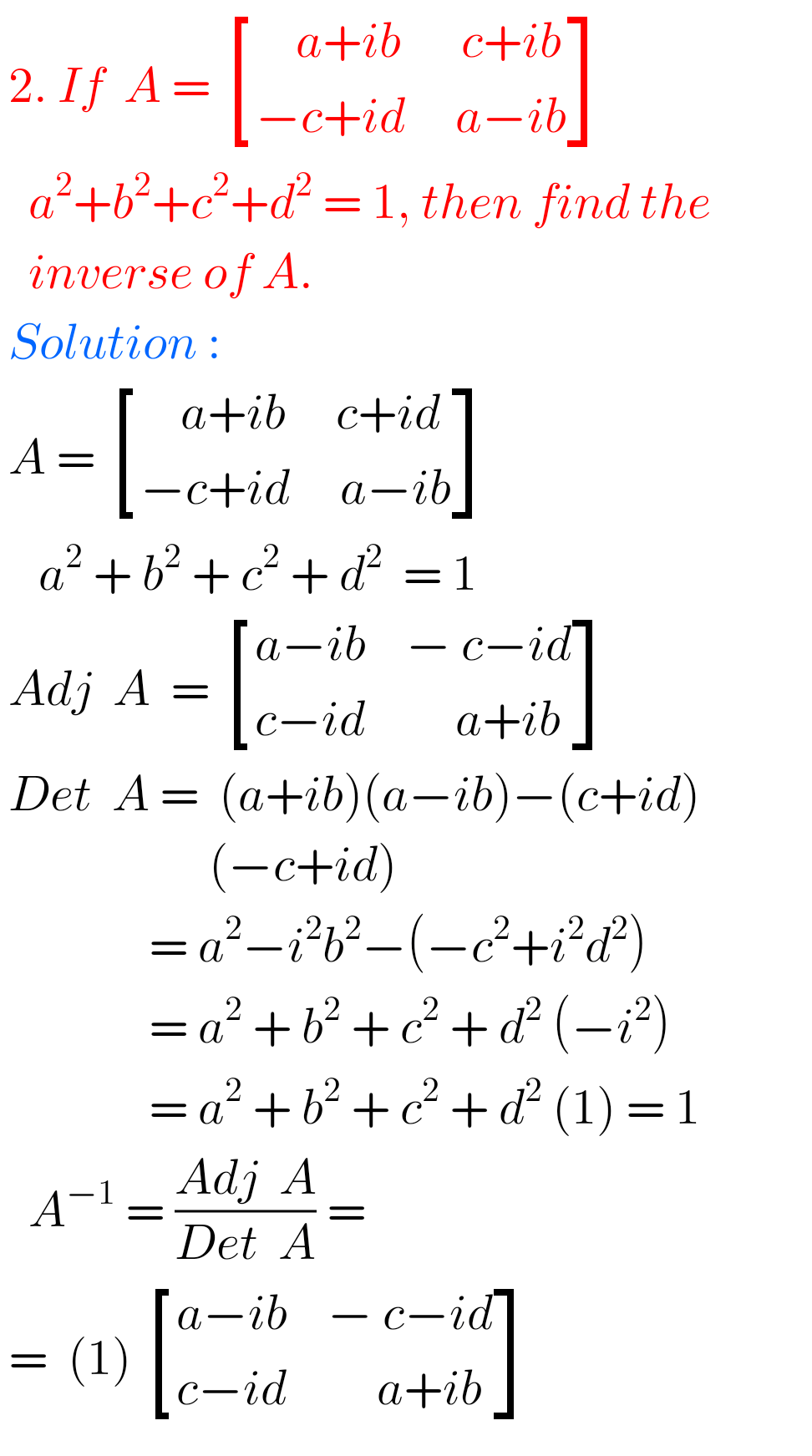## Intermediate Maths Solutions for Exercise 3(e) Matrices

Intermediate Maths solutions for Matrices Exercise 3(e) Inter Maths 1A text book Exercise 3(e) Matrices solutions are given. These solutions are very easy to understand. Please study the text book lesson Matrices very well. Observe the example problems and solutions given in the text book. Observe the given solutions and try them in your own …## Intermediate Maths Solutions for Exercise 3(d) Matrices

Intermediate Maths solutions for Matrices Exercise 3(d) Inter Maths 1A text book Exercise 3(d) Matrices solutions are given. These solutions are very easy to understand. Study the text book lesson Matrices very well.  Observe the example problems and solutions given in the text book. Observe the given solutions and try them in your own method. …## Intermediate Maths Solutions for Matrices Exercise 3(c)

Intermediate Maths solutions for Matrices Exercise 3(c) Inter Maths 1A text book Exercise 3(c) Matrices solutions are given. These solutions are very easy to understand. Please study the text book lesson Matrices very well.  Observe the example problems and solutions given in the text book. Observe the given solutions and try them in your own …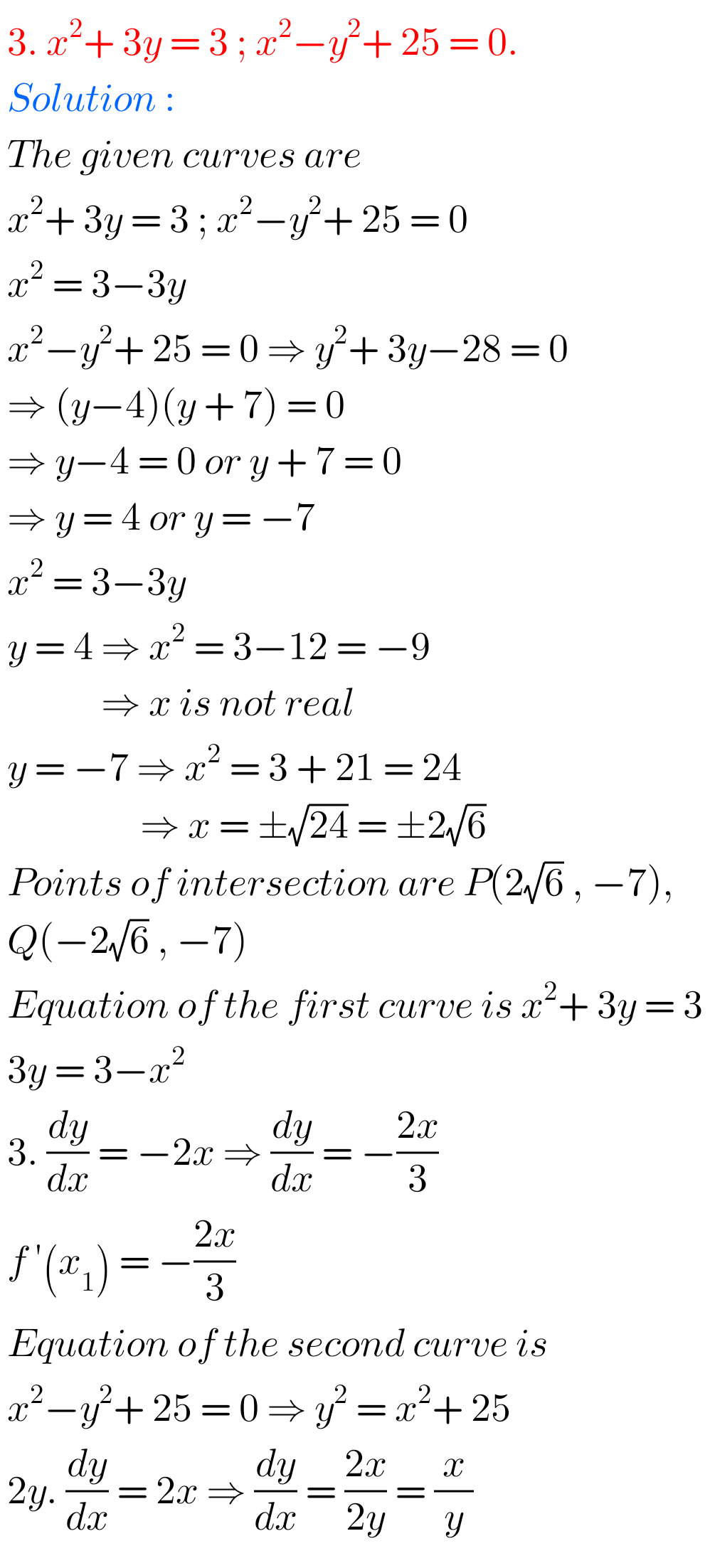## Applications of Derivatives exercise 10(d) solutions Inter

Inter Maths Solutions for Applications of Derivatives exercise 10(d) Intermediate Mathematices 1B Solutions for exercise 10(d) Applications of Derivatives are given. Study the textbook lesson Applications of Derivatives very well. Practice the example problems and solutions given in the textbook. Observe the solutions given below and try them in your own method. You can also …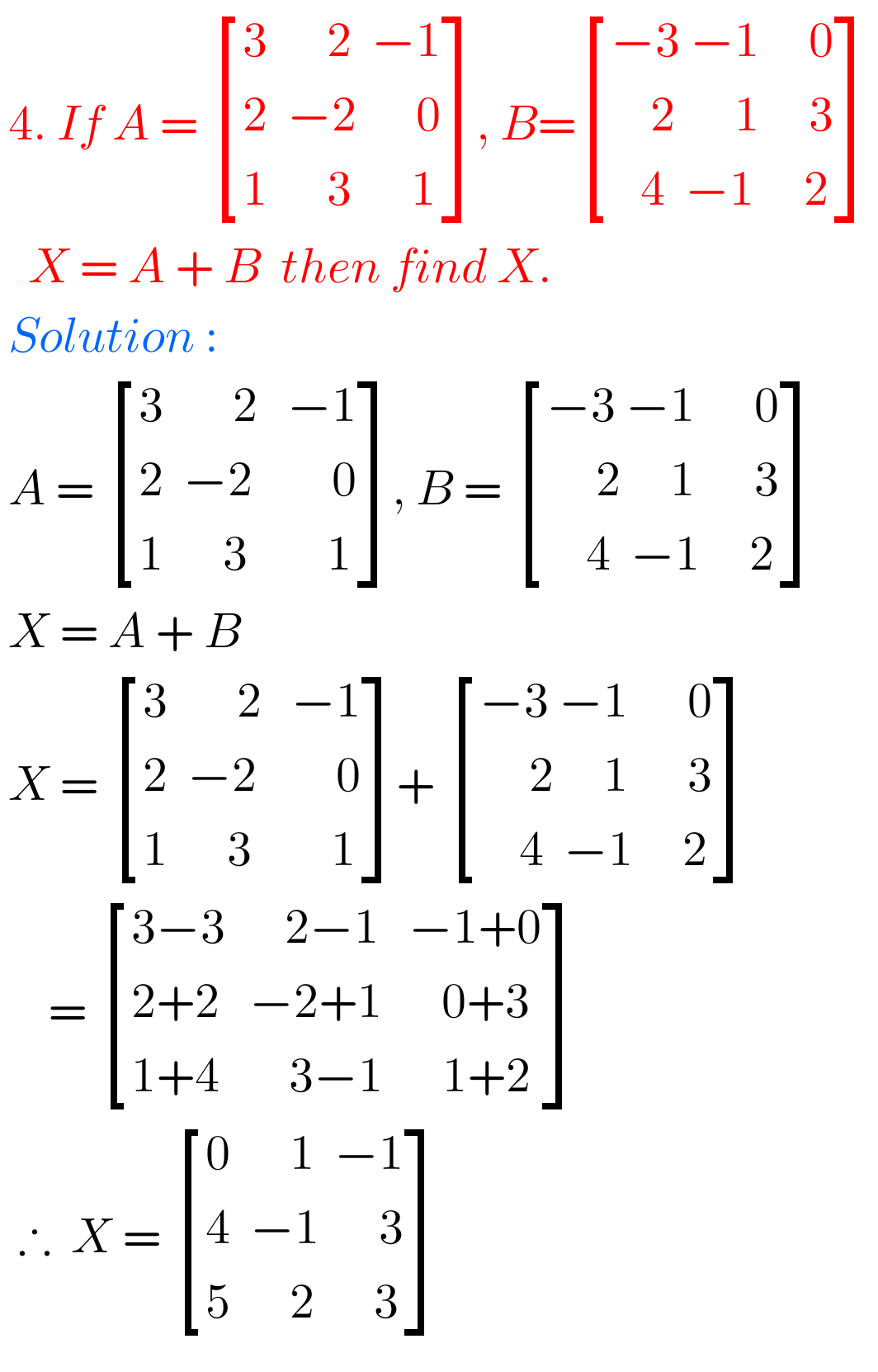## Intermediate Maths solutions for exercise 3(a) Matrices

Intermediate Maths Solutions for Exercise 3(a) Matrices Inter Maths 1A text book Exercise 3(a) Matrices solutions are given. These solutions are very easy to understand. Please study the text book lesson Matrices very well.  Observe the example problems and solutions given in the text book. Try them well. Observe the given solutions and try them …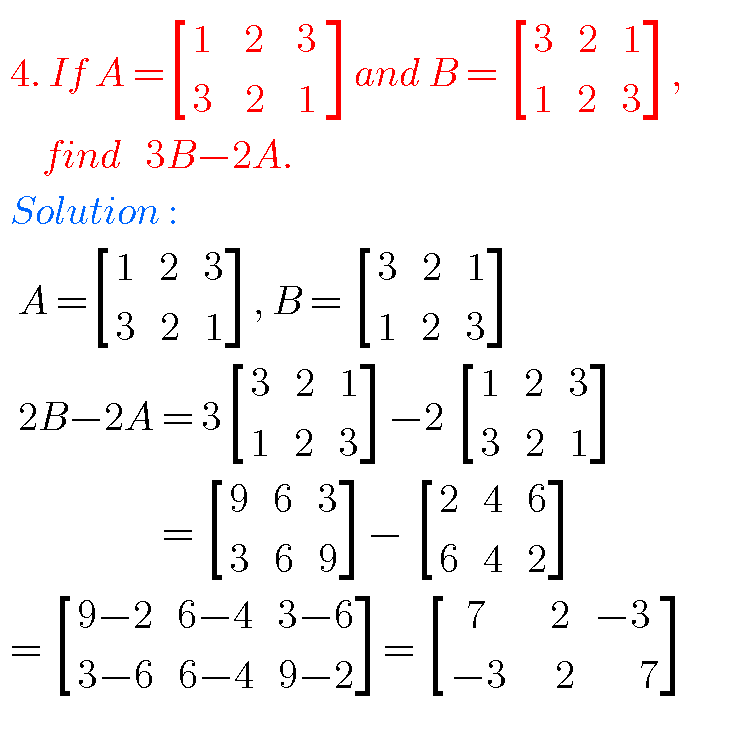## Matrices solutions,Inter Maths 1a chapter 3 solutions

Matrices solutions, Inter maths 1a chapter 3 solutions Mathematics intermediate first year 1a matrices solutions for some problems for examination purpose. Study the text book lesson Matrices very well. Practice the example problems and solutions given in the textbook. Observe the solutions and try them in your own method. You can also see Inter Maths …## Intermediate Maths Solutions for Matrices Exercise 3(g)

Intermediate Maths solutions for Matrices Exercise 3(g) Inter Maths 1A text book Exercise 3(g) Matrices solutions are given. These solutions are very easy to understand. Please study the text book lesson Matrices very well. Observe the example problems and solutions given in the text book. Try them well. Observe the given solutions and try them …## Intermediate Maths Solutions for Matrices Exercise 3(h)

Intermediate Maths solutions for Matrices Exercise 3(h) Inter Maths 1A text book Exercise 3(h) Matrices solutions are given. These solutions are very easy to understand. Please study the text book lesson Matrices very well. Observe the example problems and solutions given in the text book. Observe the given solutions and try them in your own …## Exercise 10(c) Applications of Derivatives solutions textbook Inter

Inter Maths solutions for Applications of Derivatives exercise 10(c) Intermediate Mathematices 1B Solutions for exercise 10(c) Applications of Derivatives are given. Study the textbook lesson Applications of Derivatives very well. Practice the example problems and solutions given in the textbook. Observe the solutions given below and try them in your own method. You can also …